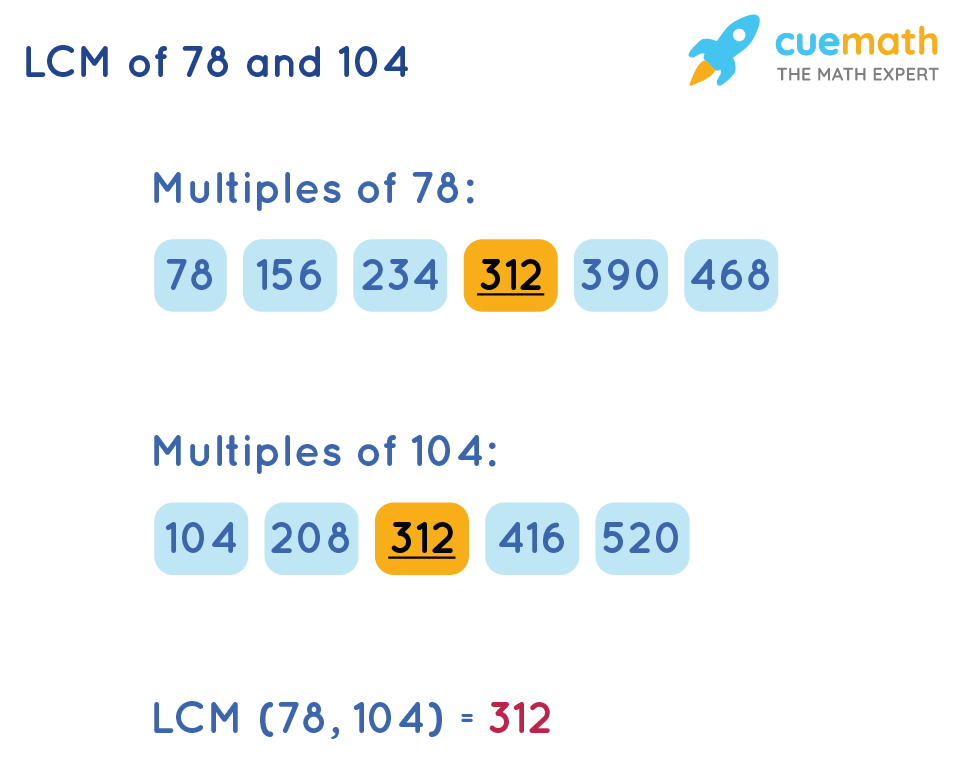# What is the LCM of 78 and 104?

## Question: What is the LCM of 78 and 104?

The LCM (Least Common Multiple) of 78 and 104 is the least number which is exactly divisible by all the numbers 78 and 104.

## Answer: LCM of 78 and 104 is 312

Look at the two methods shown below to find the LCM of 78 and 104.

## Explanation:

The two methods that we are using to find LCM(78, 104) are shown below.

• LCM of 78 and 104 by Listing the Common Factors
• LCM of 78 and 104 by Formula

### Method1: LCM of 78 and 104 by Listing Method

We will list out the first few multiples of 78 and 104 and determine the common multiples of 78 and 104.The least among the common multiples is the LCM of 78 and 104. Here, 312 is the least common multiple of 78 and 104.

### Method2: LCM of 78 and 104 by Formula

We will use the formula of LCM (a, b):

LCM (a, b) = (a × b) / HCF (a, b)

HCF (a, b) is the highest common factor of a and b.

Use this formula to find the LCM of 78 and 104.

LCM (78, 104) = (78 × 104) / HCF (78, 104)

= 8112 / 26

= 312

By using any of the above methods, the answer to LCM of 78 and 104 is the same.